# getrom

Obtain reduced-order models when using balanced truncation method

Since R2023b

## Syntax

``rsys = getrom(R,Name=Value)``
``[rsys,info] = getrom(R,___)``
``rsys = getrom(R)``
``getrom(R,"-help")``

## Description

Use `getrom` to obtain reduced-order models from a `BalancedTruncation` or `SparseBalancedTruncation` model order reduction task. For the full workflow, see Task-Based Model Order Reduction Workflow.

example

````rsys = getrom(R,Name=Value)` returns a reduced-order model `rsys` based on the options specified by one or more name-value arguments.```
````[rsys,info] = getrom(R,___)` also returns a structure `info` containing additional information, such as the left and right projector matrices.```
````rsys = getrom(R)` returns a simplified model `rsys`.For ordinary balanced truncation, `rsys` is a simplified model where all states associated with numerically zero Hankel singular values (HSVs) are removed. This amounts to a minimal realization of the original system `sys`For sparse balanced truncation, `rsys` is the reduced-order model associated with the computed HSVs. These are the (numerically nonzero) singular values of LrTLo, where Lr and Lo are the low-rank Gramian factors available in `R`. Since Lr and Lo are tall and skinny, the order of `rsys` is typically much smaller than the order of `sys`. You can further reduce the order by dropping states with relatively small HSVs.```
````getrom(R,"-help")` returns help specific to the model order specification object `R`. The returned help shows the name-value arguments and syntaxes applicable to `R`.```

## Examples

collapse all

This example shows how to create a model order reduction specification for a dense LTI model using the balanced truncation method.

For this example, generate a random discrete-time state-space model with 40 states.

```rng(0) sys = drss(40);```

Plot the Bode response.

`bode(sys)`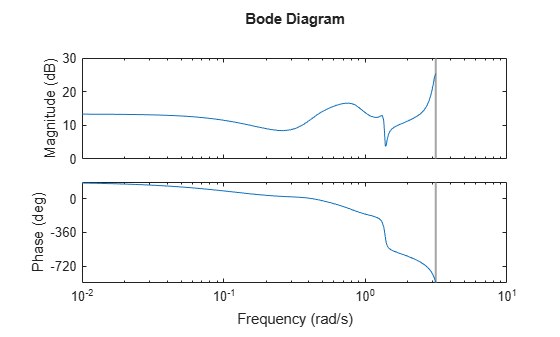Create the specification object.

`R = reducespec(sys,"balanced");`

Notice that `reducespec` does not perform any computation and creates only the object. This allows you to set additional options before running the model order reduction algorithm, such as relative error control as the algorithm objective.

`R.Options.Goal = "relative";`

For balanced truncation, you can visualize the contributions in terms of the Hankel singular values or normalized energies. By default, the `view` function plots Hankel singular values.

`view(R)`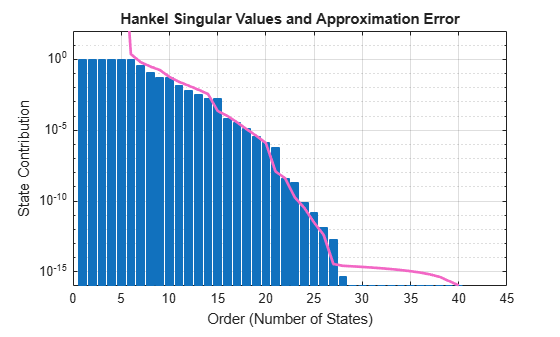For this example, select an order of 15 since it is the first order with a relative error less than `1e-4`. In general, you select the order based on the desired absolute or relative fidelity. Then, compute the reduced-order model.

`rsys = getrom(R,Order=15);`

Plot the Bode response of both models.

```bode(sys,rsys,'r--') legend("Original","Order 15")```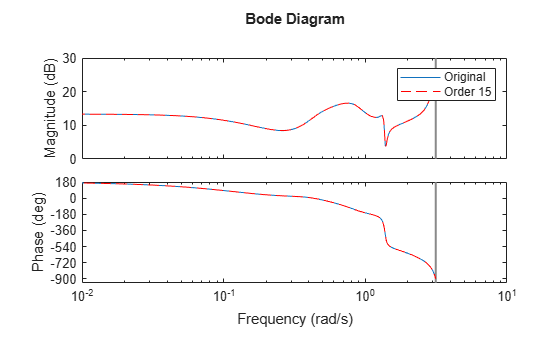This example shows how to obtain a reduced-order model for a linear time-invariant (LTI) model using the balanced truncation method. In this example, you reduce a high-order model with a focus on the dynamics in a particular frequency range.

Load a model and examine its frequency response.

```load('highOrderModel.mat','G') bodeplot(G)```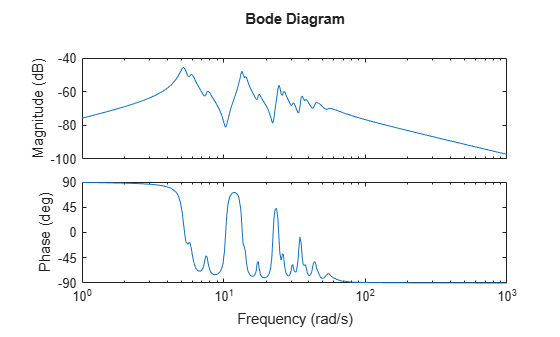`G` is a 48th-order model with several large peak regions around 5.2 rad/s, 13.5 rad/s, and 24.5 rad/s, and smaller peaks scattered across many frequencies. Suppose that for your application you are only interested in the dynamics near the second large peak, between 10 rad/s and 22 rad/s. Focus the model reduction on the region of interest to obtain a good match with a low-order approximation.

Create a model order reduction task and specify the desired frequency interval.

```R = reducespec(G,"balanced"); R.Options.FreqIntervals = [10,22];```

Analyze the model and compute the derived information.

`R = process(R);`

Use the `getrom` function to retain all the modes between 10 rad/s and 22 rad/s.

`[rsys,info] = getrom(R,Order=[10,18],Method="truncate");`

Examine the frequency responses of the reduced-order models. Also, examine the difference between those responses and the original response (the absolute error).

```subplot(2,1,1); bodemag(G,rsys(:,:,1,1),rsys(:,:,2,1),logspace(0.5,1.5,100)) title('Bode Magnitude Plot') legend("Original","Order 10", "Order 18") subplot(2,1,2); bodemag(G-rsys(:,:,1,1),G-rsys(:,:,2,1),logspace(0.5,1.5,100)); title('Absolute Error Plot') legend('Order 10','Order 18');```With the frequency-limited energy computation, even the 10th-order approximation is quite good in the region of interest.

For this example, consider the MIMO state-space model `cdrom` with 120 states. You can use absolute or relative error control when approximating models with balanced truncation. This example compares the two approaches when applied to one of the I/O channels of a 120-state model of a portable CD player device `crdom` [1,2].

Load the CD player state-space model.

```load cdromData.mat cdrom sys = cdrom(1,2); size(sys)```
```State-space model with 1 outputs, 1 inputs, and 120 states. ```

To compare absolute with relative error control, create one specification for each approach. By default, the goal of the algorithm is absolute error control.

```Rabs = reducespec(sys,"balanced") ; Rrel = reducespec(sys,"balanced"); Rrel.Options.Goal = "relative";```

Compute reduced-order models of order 15 with both approaches.

```rsys_abs = getrom(Rabs,Order=15,Method="truncate"); rsys_rel = getrom(Rrel,Order=15,Method="truncate"); size(rsys_abs)```
```State-space model with 1 outputs, 1 inputs, and 15 states. ```
`size(rsys_rel)`
```State-space model with 1 outputs, 1 inputs, and 15 states. ```

Plot the Bode response of the original model along with the absolute-error and relative-error reduced models.

```bo = bodeoptions; bo.PhaseMatching = 'on'; bodeplot(sys,rsys_abs,'r--',rsys_rel,'g-.',bo) legend("Original (120 states)","Absolute Error (15 states)",... "Relative Error (15 states)","Location","southwest")```The Bode response of:

• The relative-error reduced model `rsys_rel` nearly matches the response of the original model `sys` across the complete frequency range.

• The absolute-error reduced model `rsys_abs` matches the response of the original model `sys` only in areas with the most gain.

This example shows how you can use input and output scaling to emphasize accuracy of model order reduction in a particular channel of a MIMO model.

Load the CD player state-space model.

```load cdromData.mat cdrom size(cdrom)```
```State-space model with 2 outputs, 2 inputs, and 120 states. ```

Create a model order reduction task.

`R = reducespec(cdrom,"balanced");`

Specify the input and output weights as static values such that the (1,2) channel is dominant.

```R.Options.InputWeight = diag([1e-3 1]); R.Options.OutputWeight=diag([1 0.1]);```

Obtain the reduced-order model.

```rsys = getrom(R,MaxError=1e-2,Method="truncate"); bode(cdrom,rsys)```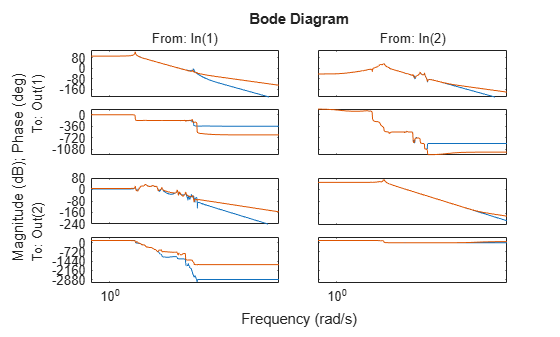The reduced-order model provides a good match for the scaled I/O channel.

Model order reduction method controls the overall error of the MIMO model, so it is unlikely you get high relative accuracy on all channels when the gains are very different. Scaling an I/O channel helps you control the accuracy for that particular channel.

This example shows how to perform balanced truncation of a sparse state-space model obtained from linearizing a thermal model of heat distribution in a circular cylindrical rod.

```load cylindricalRod.mat sys = sparss(A,B,C,D,E); size(sys)```
```Sparse state-space model with 3 outputs, 1 inputs, and 7522 states. ```

The thermal model contains 7522 states.

Create a balanced truncation specification object for `sys` and run the algorithm.

```R = reducespec(sys,"balanced"); R = process(R)```
```Initializing... Running ADI with built-in shifts......... Running ADI with adaptive shifts.. Solved Lyapunov equations to desired accuracy. ```
```R = SparseBalancedTruncation with properties: Sigma: [40x1 double] Energy: [40x1 double] Error: [40x1 double] Lr: [7522x40 double] Lo: [7522x123 double] Residuals: [6.2579e-09 8.9726e-09] Options: [1x1 mor.SparseBalancedTruncationOptions] ```

Use the `view` command to visualize the state contributions as Hankel singular values

`view(R,"sigma")`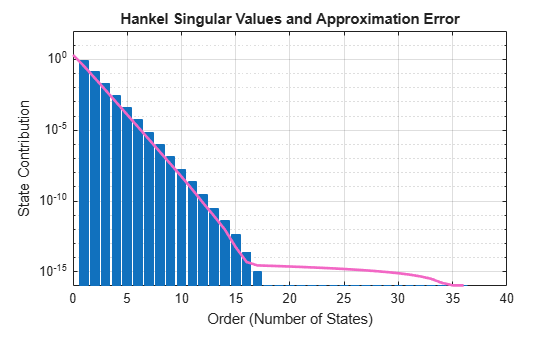Obtain the reduced-order model with maximum error of `1e-6`. This results in a model with order 8.

`rsys = getrom(R,MaxError=1e-6,Method="truncate");`

Compare the singular value response of the models.

```w = logspace(-7,-3,20); fsys = frd(sys,w); sigma(fsys,fsys-rsys,'r--')```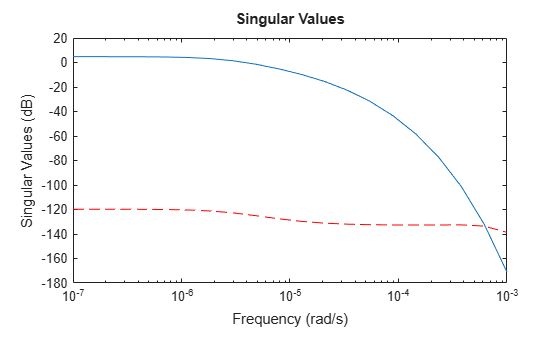The reduced-order model is a good match for the full-order model.

This example shows how to improve the balanced truncation approximation with the help of frequency weights to emphasize accuracy in a particular frequency band.

For this example, generate a random state-space model with 30 states, two outputs, and three inputs.

```rng(2) sys = rss(30,2,3); size(sys)```
```State-space model with 2 outputs, 3 inputs, and 30 states. ```

Create a model order reduction task.

`R = reducespec(sys,"balanced");`

Obtain the reduced order model.

```rsys = getrom(R,Order=19); sigma(sys,sys-rsys,"r--") legend("sys","sys-rsys")```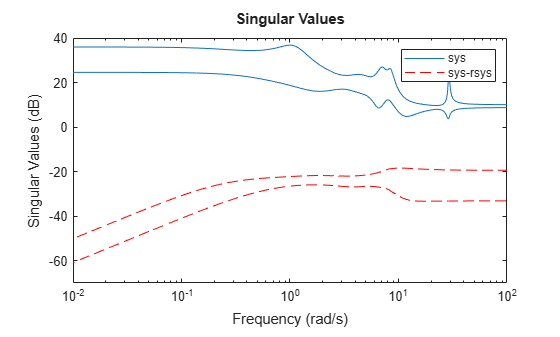This reduced-order model does not provide a great approximation in the high frequencies. To improve the response, you can use the options to specify frequency weights to emphasize accuracy in a particular frequency band. These weights should have high gain in frequency bands of interest and low gain elsewhere.

Specify the frequency weights with a high gain in the 3 rad/s to 100 rad/s band.

```wi = tf([1 0 0 0],[1 6 18 27])*eye(3); wo = tf(1,[0.01 1])*eye(2); R.Options.InputWeight = wi; R.Options.OutputWeight = wo;```

Obtain the reduced-order model.

```rsysFW = getrom(R,Order=19); sigma(sys,sys-rsys,"r--",sys-rsysFW,"k-.") legend({"sys","rsys","rsysFW"},FontSize=8)```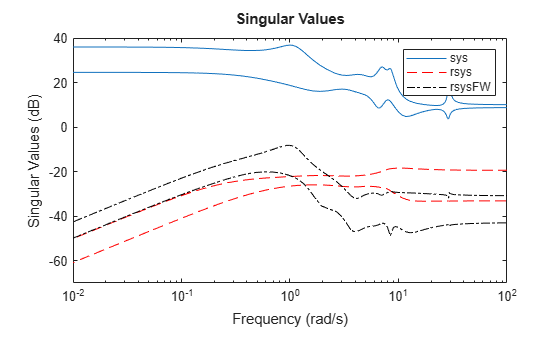The model with frequency weighted reduction provides a better approximation across the higher frequencies.

## Input Arguments

collapse all

Model order reduction specification object created using `reducespec`, specified as a `BalancedTruncation` or `SparseBalancedTruncation` object.

### Name-Value Arguments

Specify optional pairs of arguments as `Name1=Value1,...,NameN=ValueN`, where `Name` is the argument name and `Value` is the corresponding value. Name-value arguments must appear after other arguments, but the order of the pairs does not matter.

Example: `rsys = getrom(R,Order=10,Method="matchDC")`

Desired order of the reduced-order model, specified as a nonnegative scalar or vector.

• To obtain a single reduced-order model `rsys`, use a scalar.

• To obtain an array of reduced models `rsys`, use a vector.

For example, if you specify ```rsys = getrom(R,Order=[5,8,11])```, `rsys` is a 3-by-1 model array containing reduced-order models with orders of 5, 8, and 11.

The arguments `Order`, `MaxError`, and `MinEnergy` are mutually exclusive. You can specify only one of these arguments at a time, along with the optional `Method` argument.

Maximum approximation error, specified as a nonnegative scalar or vector.

• To obtain a single reduced-order model `rsys`, use a scalar.

• To obtain an array of reduced models `rsys`, use a vector.

For example, if you specify ```rsys = getrom(R,MaxError=[1e-3,1e-5,1e-7])```, `rsys` is a 3-by-1 model array containing reduced-order models with the specified error bounds.

The function selects the lowest order for which the error does not exceed the value you specify for this argument.

The arguments `Order`, `MaxError`, and `MinEnergy` are mutually exclusive. You can specify only one of these arguments at a time, along with the optional `Method` argument.

Minimum bound on the normalized energy, specified as a nonnegative scalar or vector.

• To obtain a single reduced-order model `rsys`, use a scalar.

• To obtain an array of reduced models `rsys`, use a vector.

For example, if you specify ```rsys = getrom(R,MinEnergy=[1e-3,1e-5])```, `rsys` is a 2-by-1 model array containing reduced-order models with the specified minimum normalized energies of the states.

The function discards all the states that have normalized energies lower than the value you specify for this argument.

The arguments `Order`, `MaxError`, and `MinEnergy` are mutually exclusive. You can specify only one of these arguments at-a time, along with the optional `Method` argument.

State elimination method, specified as `"matchDC"` or `"truncate"`. This argument specifies how the function eliminates the states with weak contribution.

• The `"matchDC"` method preserves the DC gain (steady-state value of step response) and tends to match the time response better.

• The `"truncate"` method tends to match the frequency response better. Use this method when you want to control the relative error.

## Output Arguments

collapse all

Reduced-order model, returned as a state-space model or an array of state-space models.

Additional information about the reduced-order model, returned as a structure or structure array.

• If `rsys` is a single state-space model, then `info` is a structure.

• If `rsys` is an array of state-space models, then `info` is a structure array with the same dimensions as `rsys`.

Each `info` structure has these fields:

FieldDescription
`PL`

Left projector matrix, returned as a matrix with dimensions:

• Nx-by-Nxr (`"truncate"`)

• Nx-by-Nnz (`"matchDC"`)

Here, Nx is the number of states in the original full-size model, Nxr is the number of states in the reduced-order model, and Nnz is the number of nonzero HSVs. In the sparse case, the software treats non-computed HSVs as zero.

`PR`

Right projector matrix, returned as a matrix with dimensions:

• Nx-by-Nxr (`"truncate"`)

• Nx-by-Nnz (`"matchDC"`)

Here, Nx is the number of states in the original full-size model, Nxr is the number of states in the reduced-order model, and Nnz is the number of nonzero HSVs. In the sparse case, the software treats non-computed HSVs as zero.

collapse all

### Spectral Projector Matrices

The function also returns the left and right spectral projector matrices in the `info` argument. When you use `Method` = `"truncate"`, the matrices Ar, Br, Cr, Dr, and Er of the reduced-order model are related to the matrices A, B, C, D, and E of the original model by:

• For explicit state-space models

`$\begin{array}{cccc}{A}_{r}={P}_{L}^{T}A{P}_{R},& {B}_{r}={P}_{L}^{T}B,& {C}_{r}=C{P}_{r},& {E}_{r}={P}_{L}^{T}{P}_{R}=I\end{array}$`
• For descriptor state-space models

`$\begin{array}{cccc}{A}_{r}={P}_{L}^{T}A{P}_{R},& {B}_{r}={P}_{L}^{T}B,& {C}_{r}=C{P}_{r},& {E}_{r}={P}_{L}^{T}E{P}_{R}\end{array}$`

When you use `Method` = `"matchDC"`, the projectors give the matrices

`$\begin{array}{c}{P}_{L}^{T}A{P}_{R}=\left[\begin{array}{cc}{A}_{11}& {A}_{12}\\ {A}_{21}& {A}_{22}\end{array}\right],\\ {P}_{L}^{T}B=\left[\begin{array}{c}{B}_{1}\\ {B}_{2}\end{array}\right],\\ C{P}_{R}=\left[\begin{array}{cc}{C}_{1}& {C}_{2}\end{array}\right],\\ {P}_{L}^{T}E{P}_{R}=\left[\begin{array}{cc}{E}_{11}& 0\\ 0& {E}_{22}\end{array}\right],\end{array}$`

and the function obtains the reduced-order model in differential algebraic equation (DAE) form by setting 2 = 0, or equivalently E22 = 0 (see `xelim`).

 Benner, Peter, Jing-Rebecca Li, and Thilo Penzl. “Numerical Solution of Large-Scale Lyapunov Equations, Riccati Equations, and Linear-Quadratic Optimal Control Problems.” Numerical Linear Algebra with Applications 15, no. 9 (November 2008): 755–77. https://doi.org/10.1002/nla.622.

 Benner, Peter, Martin Köhler, and Jens Saak. “Matrix Equations, Sparse Solvers: M-M.E.S.S.-2.0.1—Philosophy, Features, and Application for (Parametric) Model Order Reduction.” In Model Reduction of Complex Dynamical Systems, edited by Peter Benner, Tobias Breiten, Heike Faßbender, Michael Hinze, Tatjana Stykel, and Ralf Zimmermann, 171:369–92. Cham: Springer International Publishing, 2021. https://doi.org/10.1007/978-3-030-72983-7_18.

 Varga, A. “Balancing Free Square-Root Algorithm for Computing Singular Perturbation Approximations.” In  Proceedings of the 30th IEEE Conference on Decision and Control, 1062–65. Brighton, UK: IEEE, 1991. https://doi.org/10.1109/CDC.1991.261486.

 Green, M. “A Relative Error Bound for Balanced Stochastic Truncation.” IEEE Transactions on Automatic Control 33, no. 10 (October 1988): 961–65. https://doi.org/10.1109/9.7255.